Question

# 1. Convolution of Discrete Time Signals Analytically determine the convolutions: (a) Find yn]-nun], where un] is...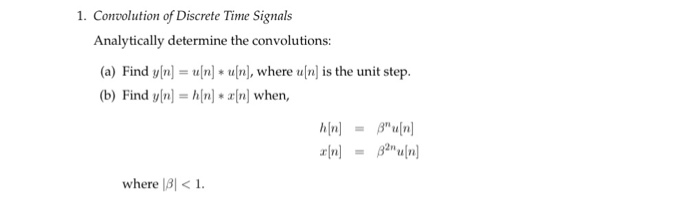1. Convolution of Discrete Time Signals Analytically determine the convolutions: (a) Find yn]-nun], where un] is the unit step (b) Find yln] z[n] when, where 131 <1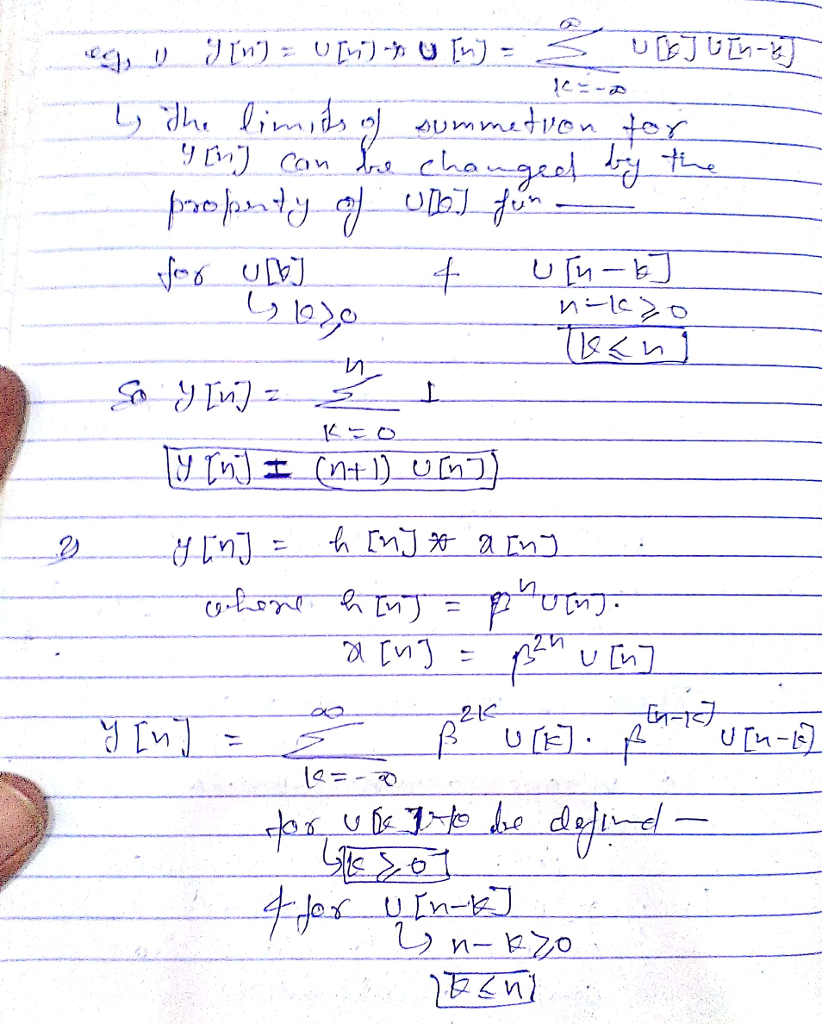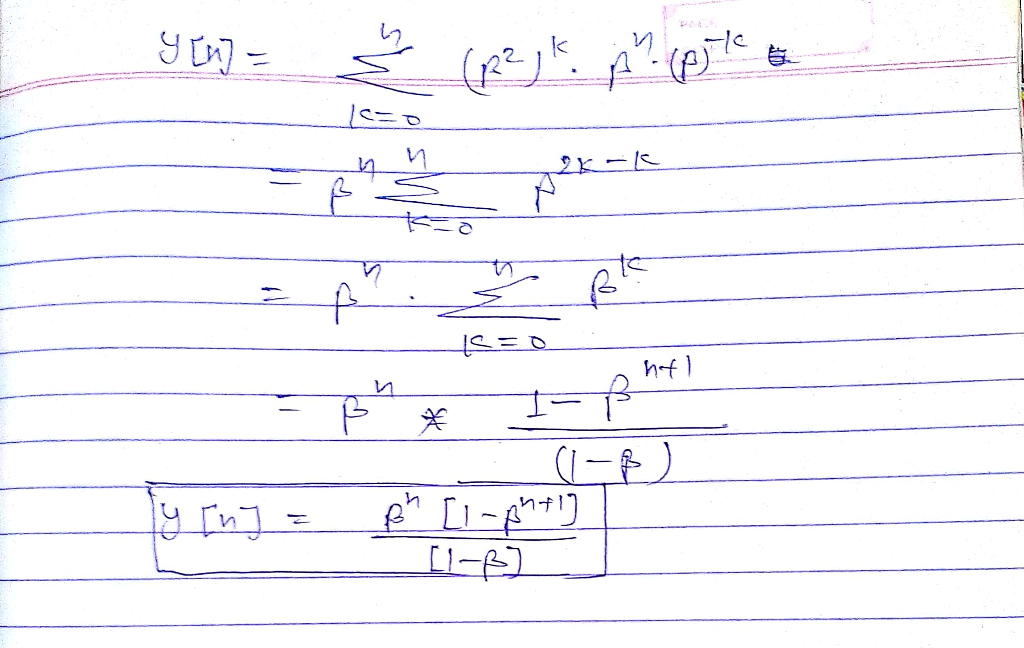#### Earn Coins

Coins can be redeemed for fabulous gifts.

Similar Homework Help Questions
• ### Consider a discrete-time LTI system with impulse response hn on-un-1), where jal < 1. Find the...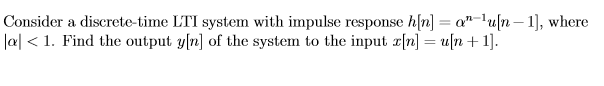Consider a discrete-time LTI system with impulse response hn on-un-1), where jal < 1. Find the output y[n] of the system to the input x[n] = un +1].

• ### solve with steps and please write as clear as possible. Determine, analytically, the convolution y(t)-r(t) *...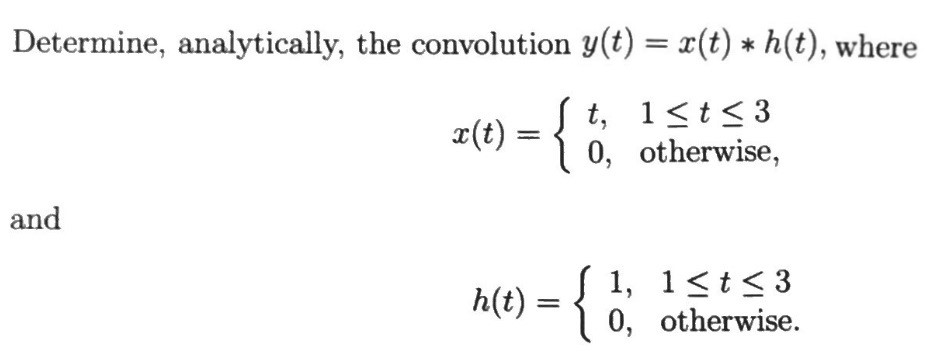solve with steps and please write as clear as possible. Determine, analytically, the convolution y(t)-r(t) * h(t), where a(t)0, otherwise, and h(t) 1, 1<t < 3 o, otherwise.

• ### QUESTION THREE With the aid of a diagram define impulse response fully using correct 141 a) Notation 141 Find the impulse response of a discrete time accumulator b) 15) c) Derive the convolution...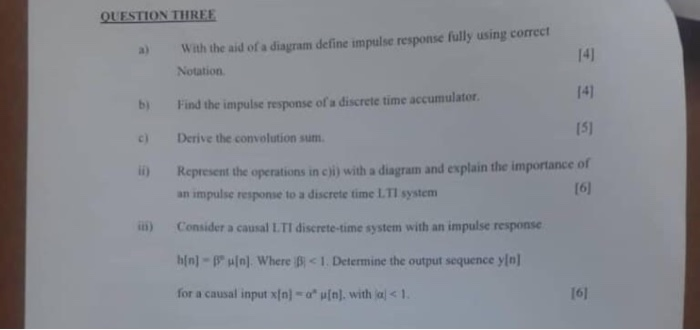QUESTION THREE With the aid of a diagram define impulse response fully using correct 141 a) Notation 141 Find the impulse response of a discrete time accumulator b) 15) c) Derive the convolution sum esent the operations in cji) with a diagram and explain the importance of an impulse response to a discrete time L.TI system 16) in) Consider a causal L.TI discrete-time system with an impulse response h1n1-r 비nl where pct Determine the output sequence yln] 161 for a...

• ### 1. Discrete Fourier Analysis. Given the following periodic function: 50. 0<t<2s a) Find discrete time rot...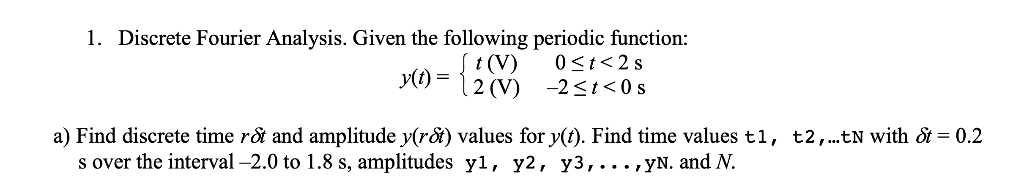1. Discrete Fourier Analysis. Given the following periodic function: 50. 0<t<2s a) Find discrete time rot and amplitude y(rSi) values for y(t). Find time values t1, t2, ..tN with ot-0.2 s over the interval -2.0 to 1.8 s, amplitudes yl, y2, y3,.. .,yN. and N.

• ### 2. Determine whether the following discrete-time signals are periodic or not? For the periodic ones, find...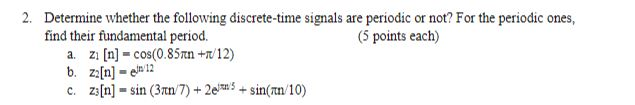2. Determine whether the following discrete-time signals are periodic or not? For the periodic ones, find their fundamental period. (5 points each) n12 c. z[n] sin (3rn7)2sin(n/10)

• ### elsewhere elsewhere 6. Convolution between two discrete time signals, #1 [n] and 12[n] is given by:...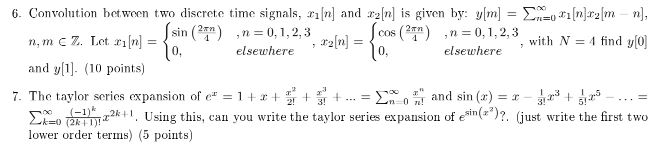elsewhere elsewhere 6. Convolution between two discrete time signals, #1 [n] and 12[n] is given by: y[m] = \$R=0[n] ru[m sin (Pan) ,n=0,1,2,3 cos (27) ,n=0,1,2,3 n, m 7. Let 21 [n] 10. with N = 4 find y(0) and y. (10 points) 7. The taylor series expansion of c" = = 1+++++ and sin(x) = 1 - 3 +420 ko 15x24. Using this, can you write the taylor series expansion of esin(x”)? (just write the first two lower order...

• ### Determine whether the following discrete-time signals are periodic or not? For the periodic ones, find their...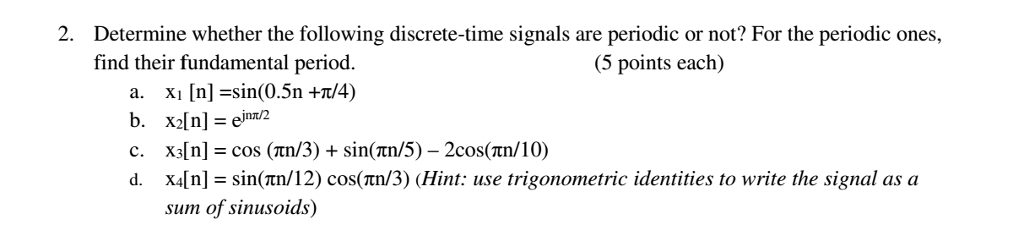Determine whether the following discrete-time signals are periodic or not? For the periodic ones, find their fundamental period. 2. (5 points each) a. x1 [n] =sin(0.5n +z/4) c. x1n] = cos (tn/3) + sin(nn/5)-2cos(tn/10) d. x4[n]- sin(tn/12) cos(tn/3) (Hint: use trigonometric identities to write the signal as a sum of sinusoids)

• ### Consider the discrete-time signal given below. Ş ()", n20 X = 0 n < 0 where...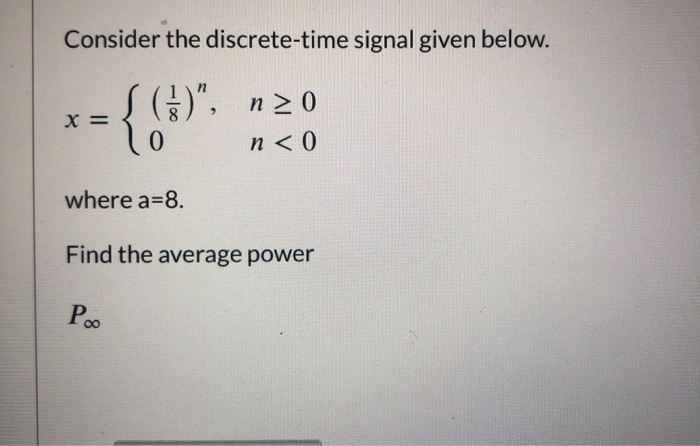Consider the discrete-time signal given below. Ş ()", n20 X = 0 n < 0 where a=8. Find the average power Poo

• ### Digital Signal Processing (EEC 4963) Assignment 1 Discrete-Time Signals Express the signal 1. 1, 3sns-1 71 elsewher...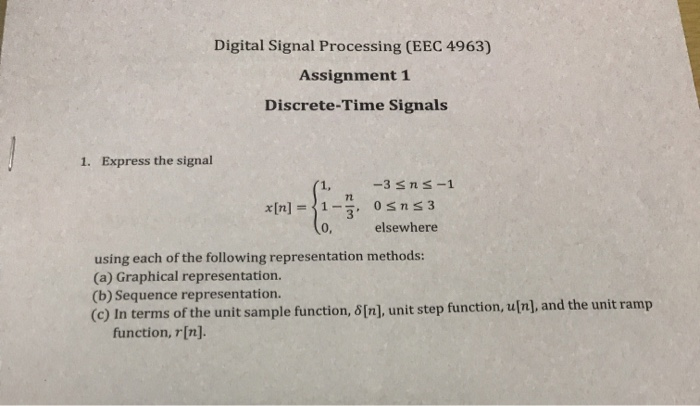Digital Signal Processing (EEC 4963) Assignment 1 Discrete-Time Signals Express the signal 1. 1, 3sns-1 71 elsewhere 0, using each of the following representation methods (a) Graphical representation. (b) Sequence representation. (c) In terms of the unit sample function, δ[n], unit step function, u[n], and the unit ramp function, r[n]. Digital Signal Processing (EEC 4963) Assignment 1 Discrete-Time Signals Express the signal 1. 1, 3sns-1 71 elsewhere 0, using each of the following representation methods (a) Graphical representation. (b) Sequence...

• ### x[n] = { Consider the discrete sequence S (0.5)" 0<n<N-1 otherwise a) Determine the z-transform X(2)!...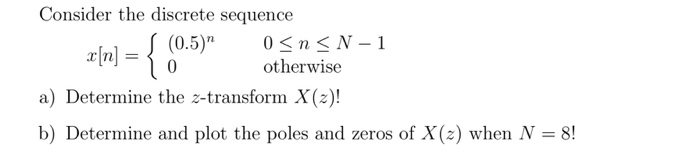x[n] = { Consider the discrete sequence S (0.5)" 0<n<N-1 otherwise a) Determine the z-transform X(2)! b) Determine and plot the poles and zeros of X(2) when N = 8!